Important Questions: Mensuration

# Important Questions: Mensuration Notes | Study Mathematics (Maths) Class 8 - Class 8

## Document Description: Important Questions: Mensuration for Class 8 2022 is part of Mensuration for Mathematics (Maths) Class 8 preparation. The notes and questions for Important Questions: Mensuration have been prepared according to the Class 8 exam syllabus. Information about Important Questions: Mensuration covers topics like and Important Questions: Mensuration Example, for Class 8 2022 Exam. Find important definitions, questions, notes, meanings, examples, exercises and tests below for Important Questions: Mensuration.

Introduction of Important Questions: Mensuration in English is available as part of our Mathematics (Maths) Class 8 for Class 8 & Important Questions: Mensuration in Hindi for Mathematics (Maths) Class 8 course. Download more important topics related with Mensuration , notes, lectures and mock test series for Class 8 Exam by signing up for free. Class 8: Important Questions: Mensuration Notes | Study Mathematics (Maths) Class 8 - Class 8
 1 Crore+ students have signed up on EduRev. Have you?

Question 1: Find the area of the following trapezium.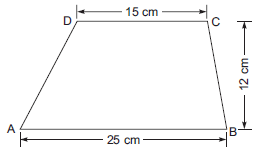Solution: Here, parallel sides are 25 cm and 15 cm.
Height (i.e. distance between the parallel sides) = 12 cm

∵ Area of a trapezium  ABCD = 1/2 x (Sum of the parallel sides) * Height

∴ Area of the trapezium ABCD

= 1/2 (25 + 15) x 12 cm

= 1/2 x 40 x 12 cm2 = 240 cm2

Thus, the required area of the trapezium ABCD = 240 cm2

Question 2: Find the length of the diagonal BD when, the area of the quadrilateral is 32 cm2.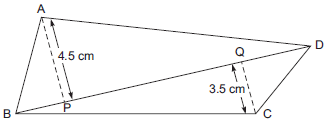Solution: Let the length of the diagonal BD = x cm
Area of the quadrilateral ABCD = 32 cm2
∵ Area of a quadrilateral = (1/2)* (A diagonal) * (Sum of the length of the perpendiculars on the
diagonal from the opposite vertices)

ABCD = 1/2 x BD x (AP + CQ)

= 1/2 * x * (4.5 +3.5)

= 1/2 * x * 8 cm2

Since area of the quadrilateral ABCD = 32 cm2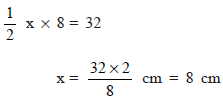Thus, the required length of the diagonal BD = 8 cm.

Question 3: The area of a rhombus and that of a square are equal. The side of the square is 6 cm. If one of the diagonal of the rhombus is 4 cm, then find the length of its other diagonal.
Solution: Here, side of the square = 6 cm
∴                             Area of the square = Side * Side
= 6 cm * 6 cm
= 36 cm2
Since,               [Area of the rhombus] = [Area of the square]
∴                         Area of the rhombus = 36 cm2
One of the diagonal of the rhombus = 4 cm
Let the other diagonal of the rhombus = d cm
∵                            Area of a rhombus = (1/2)* Product of the diagonals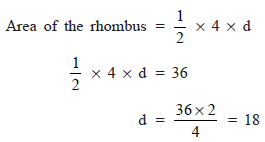Thus, the required length of the rhombus = 18 cm.

Question 4: The edge of a cube is 2 cm. Find the total surface area of the cuboid formed by three such cubes joined edge to edge.
Solution: The edge (side) of the given cube = 2 cm
Since, three such cubes are joined, then
Total length (l) = (2 + 2 + 2) cm = 6 cm
Height (h) = 2 cm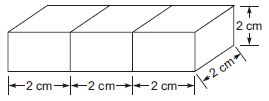∴ Total surface area of the resultant cuboid
= 2[lb + bh + hl]
= 2[6 * 2 + 2 * 2 + 2 * 6] cm2
= 2[12 + 4 + 12] cm2
= 2 cm2 = 56 cm2
Thus, the required total surface area of the cuboid = 56 cm2.

Question 5: A closed cylindrical tank of radius 7 cm and height 5 m is made from a sheet of metal.
If the breadth of the rectangular sheet is 10 m, then find the length of the sheet.
Solution: Here, Radius (r) = 7 cm
Height (h) = 5 cm
Since total surface area of the closed cylinder = 2πr (r + h)
∴  Total surface area of the cylindrical tank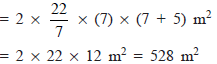Let, length of the sheet required = ‘l’ m
∴ Area of the rectangular sheet = l * 10 m2
⇒                                      l * 10 = 528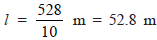Thus, the required length of the sheet = 52.8 m.

Question 6: A road roller makes 250 complete revolutions to move once over to level a road. Find the area of the road levelled if the diameter of the road roller is 84 cm and the length is 1 m.
Solution: A road roller is a cylinder, such that
Radius  =84/2 cm = 42 cm
Length (h) = 1m = 100 cm
∵ Lateral surface area of a cylinder = 2πrh
∴ Lateral surface area of the road roller
= 2 x 22/7 x 42 x 100 cm2
= 2 * 22 * 6 * 100 cm2
∴ Area of road levelled in 1 revolution = 26400 cm2
⇒ Area of road levelled in 250 revolutions
= 250 * 26400 cm2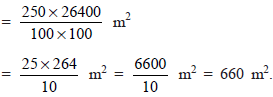Question 7: A cuboid is of dimensions 50 cm * 45 cm * 30 cm. How many small cubes with sides 5 cm can be placed in the given cuboid?
Solution: Volume of a cuboid = Length * Breadth * Height
Here,      Length of the cuboid = 50 cm
Breadth of the cuboid = 45 cm
Height of the cuboid = 30 cm
∴             Volume of the cuboid = 50 * 45 * 30 cm3
Volume of the small cube = Side * Side * Side
= 5 * 5 * 5 cm3
Let the number of the required small cubes = x
∴                           x * (5 * 5 * 5) = 50 * 45 * 30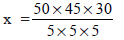= 10 * 9 * 6 = 540
Thus, 540 small cubes can be placed in the given cuboid.

The document Important Questions: Mensuration Notes | Study Mathematics (Maths) Class 8 - Class 8 is a part of the Class 8 Course Mathematics (Maths) Class 8.
All you need of Class 8 at this link: Class 8

## Mathematics (Maths) Class 8

187 videos|321 docs|48 tests
 Use Code STAYHOME200 and get INR 200 additional OFF

## Mathematics (Maths) Class 8

187 videos|321 docs|48 tests

Track your progress, build streaks, highlight & save important lessons and more!

,

,

,

,

,

,

,

,

,

,

,

,

,

,

,

,

,

,

,

,

,

;